Version 5 (modified by sam, 9 years ago) (diff)

--

# Trigonometric functions

Our research notes about implementation of fast trigonometric functions.

## Minimax polynomial for sin(x)

### Absolute error

Suppose we want to approximate sin(x) on [-π/2; π/2] with a polynomial P(x) such that the absolute error is never more than E: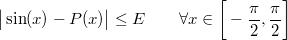We know sin(x) is an odd function, so instead we look for a polynomial Q(x) such that P(x) = xQ(x²):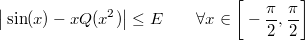Substitute y for x² and reduce the range to positive values: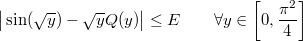Divide through by √y:If we want to force the asymptotic behaviour in x=0, we substitute Q(y) with 1+yR(y):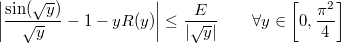Divide through by y: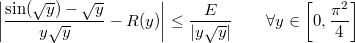We then use the following code:

static real myfun(real const &y)
{
real x = sqrt(y);
return (sin(x) - x) / (x * y);
}

static real myerr(real const &y)
{
return re(y * sqrt(y));
}

RemezSolver<6> solver;
solver.Run(real::R_1 >> 400, real::R_PI_2 * real::R_PI_2, myfun, myerr, 15);


These are the resulting R, Q and P: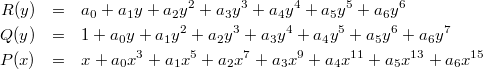With the following coefficients:

a0 = -1.66666666666658080941942898789420724e-1;
a1 = +8.33333333326271609442503773834687308e-3;
a2 = -1.98412698200591143928364634696492885e-4;
a3 = +2.75573160733868922065738227278330896e-6;
a4 = -2.50518513021429359590028300127165228e-8;
a5 = +1.60472959182597740337401201006549498e-10;
a6 = -7.36458957326227991327065122848667046e-13;